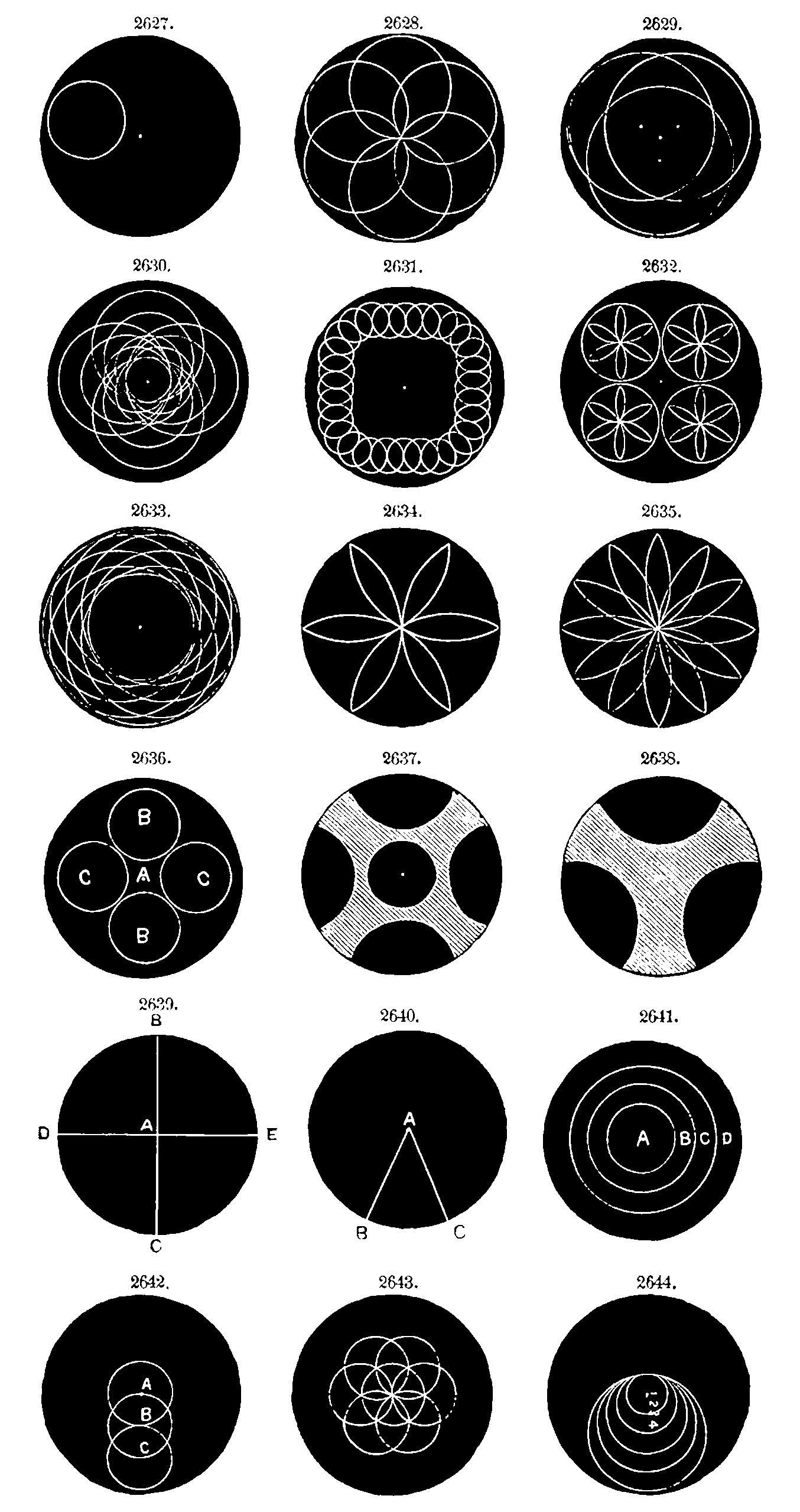# Matrix-valued random variatesDistributions who support is a random matrix. There are many of these, surely? We generally care about a small subset of possible random matrices.

The most common matrix RV distributions I see are over positive-definite matrices in particular, which can be valid covariance functions We also look at rotation matrices and matrices with i.i.d. elements.

## “Random matrices”

Despite the general-sounding name, this is frequently used for a specific degenerate case, where the elements are i.i.d. random. See random matrices.

## LKJ

Probability distribution for positive definite correlation matrices, or in practice, for their Cholesky factors.

## Matrix Gaussian

Should look them up in Gupta and Nagar (1999).

## Matrix Gamma

Currently handled under gamma processes.

## Random rotations

See random rotations.

## Matrix-F

Also introduced in Stephen R. Martin, Is the LKJ(1) prior uniform? “Yes”.

## Matrix Beta/Dirichlet

The two wikipedia summaries are sparse:

Should look them up in Gupta and Nagar (1999).

### No comments yet. Why not leave one?

GitHub-flavored Markdown & a sane subset of HTML is supported.